• 使用matlab 读取展示3D模型必备软件！！！！matlab stl
• [MATLAB学习笔记]MATLAB读取UG三维模型数据

万次阅读 多人点赞 2018-03-25 16:26:35
UG完成三维建模之后，导出STL格式的外形数据。missile.txt文件网盘链接 https://pan.baidu.com/s/1HAsXYD8bz-5MA2an6P-skg 密码: ttbxSTL格式规定模型由三个顶点组成的小三角形面片，多个面片构成了各种形状的曲面...

UG完成三维建模之后，导出STL格式的外形数据。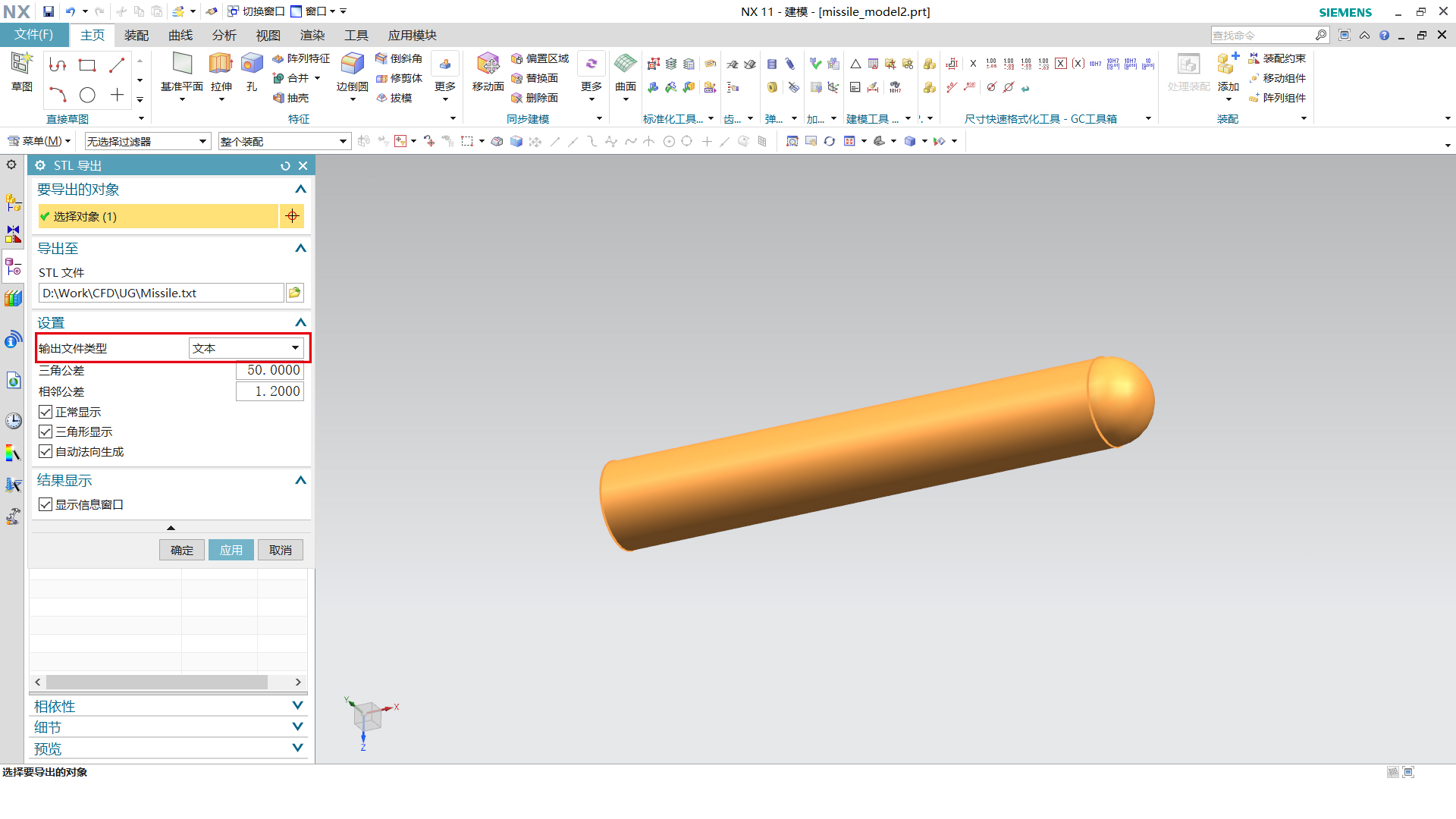missile.txt文件网盘链接

STL格式规定模型由三个顶点组成的小三角形面片，多个面片构成了各种形状的曲面。每个面片的数据采用以下格式给出

facet normal +9.9645872E-01 +8.4050291E-02 -2.3582236E-03
outer loop
vertex   +1.2993712E+03 +1.1196447E+01 +0.0000000E+00
vertex   +1.2998426E+03 +5.6070447E+00 +0.0000000E+00
vertex   +1.2998426E+03 +5.5982238E+00 -3.1438950E-01
endloop

endfacet

根据这一格式可以利用MATLAB读取模型的外形数据。

clear
clc

fid = fopen('missile.txt','r');

strline = cell(1,1);
counter = 1;
while ~feof(fid)
strline{counter,1} = fgetl(fid);
counter = counter + 1;
end
fclose(fid);
counterf = 1;
counterv = 1;
facetN = zeros(1,1);
vertex = zeros(1,1);
for n = 1:length(strline)
if length(strline{n}) == 59
if strcmp(strline{n}(3:14),'facet normal')
facetN(counterf,1) = str2double(strline{n}(16:29));
facetN(counterf,2)  = str2double(strline{n}(31:44));
facetN(counterf,3)  = str2double(strline{n}(46:59));
counterf = counterf + 1;
elseif strcmp(strline{n}(7:12),'vertex')
vertex(counterv,1) = str2double(strline{n}(16:29));
vertex(counterv,2) = str2double(strline{n}(31:44));
vertex(counterv,3) = str2double(strline{n}(46:59));
counterv = counterv + 1;
end
end
end
figure
hold on
for n = 1:length(facetN)
fill3(vertex(3*n-2:3*n,1),vertex(3*n-2:3*n,2),vertex(3*n-2:3*n,3),'y',...
'EdgeAlpha',0.5)
end
axis equal
box on

以某一导弹模型为例

UG中模型显示如图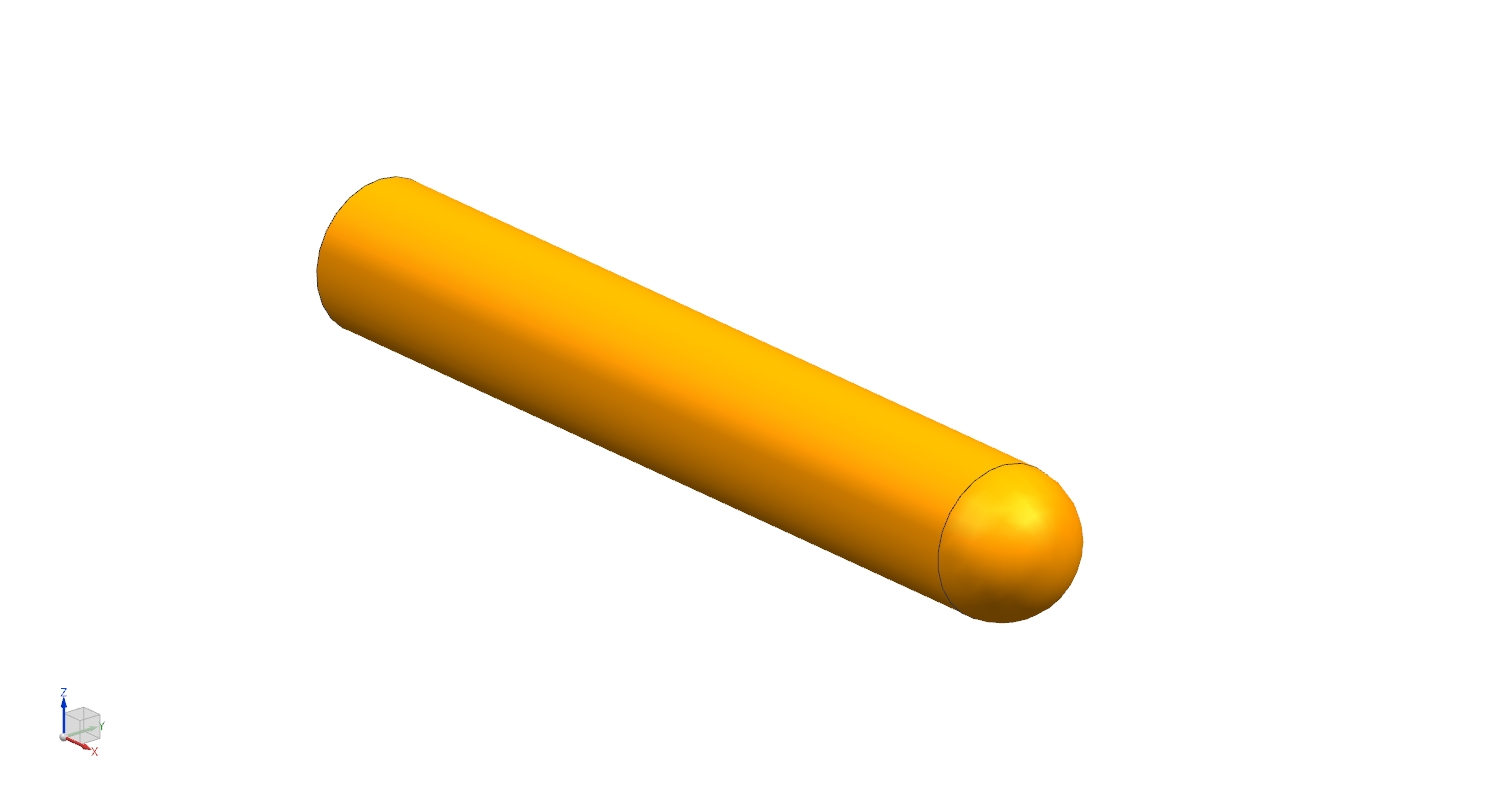导出STL格式的missile.txt的外形数据，利用MATLAB读取显示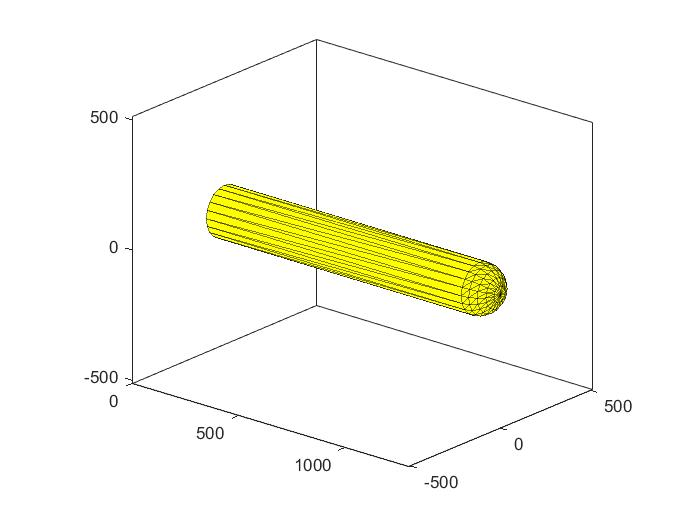展开全文MATLAB UG
• 1.STL格式 MATLAB读取UG三维模型数据 Matlab与UG/Pro E 之间的数据交换方法 将matlab程序生成的曲线导入solidworks中并生成实体
展开全文matlab
• solidworks三维模型导入MATLAB仿真操作步骤

万次阅读 多人点赞 2019-09-30 09:45:54

2 建立好solidworks三维模型

在这里我就用早期建立好的一个模型，一个智能遥控车，丑是丑了点，莫建议，反正在这里不重要。如图所示：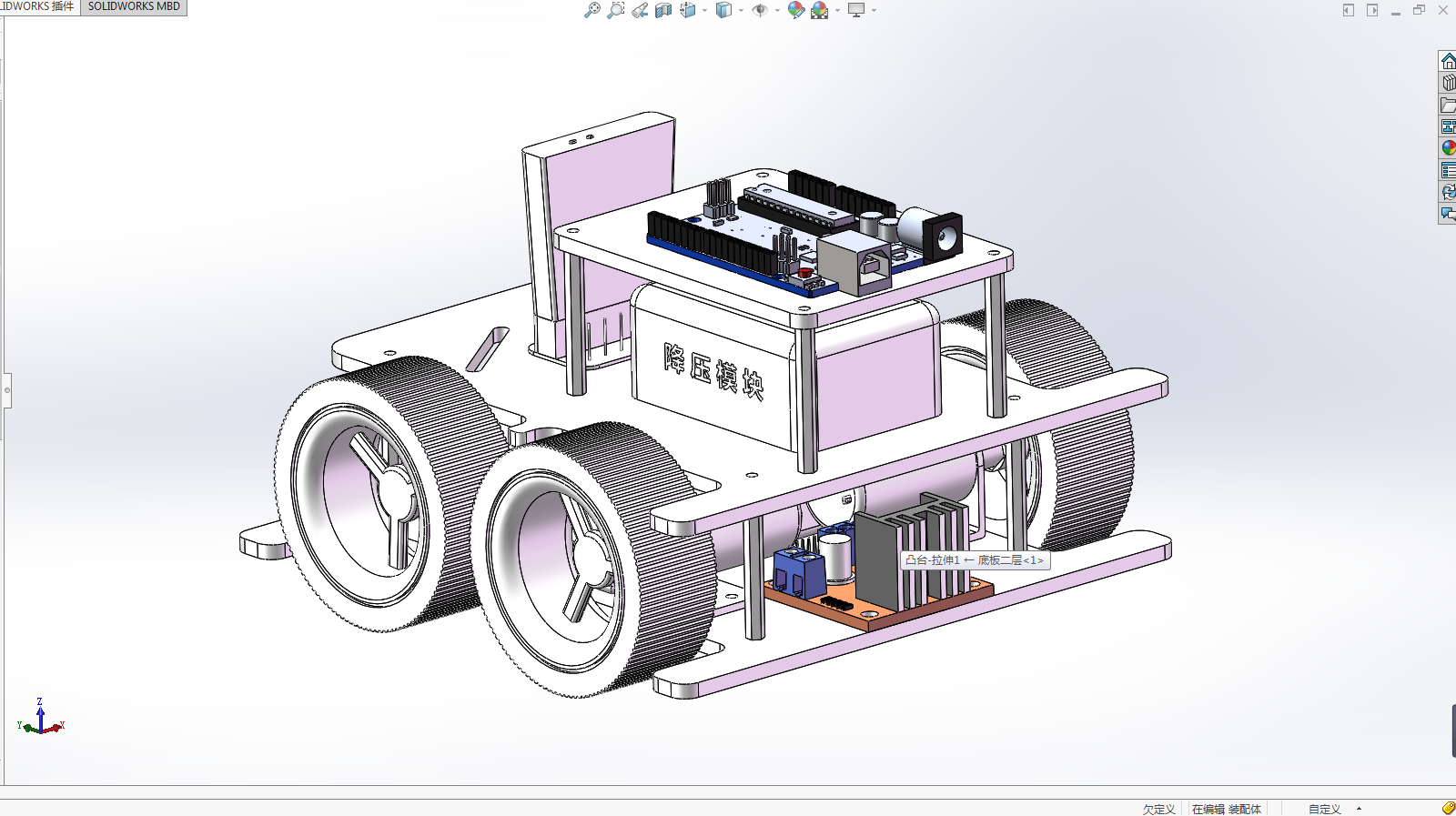其实这里也没得什么要说明的，牵强着还是写点什么，就是在导出1G和2G后在MATLAB里面运行不是一样，如此我就两个都操作一下。

3.1 1G操作方法

出现下面画面说明已经搞定，注意，在导出之前要记得设置保存路径哦，然后你看文件夹里面的内容。

然后就是打开你的MATLAB，操作跟我下面一直就行了：在这个过程，由于建立的模型零件数量大，这是会影响等待时间的，只有慢慢等。

做到这里其实有点后悔了，不该使用这个模型来做，导出的模型文件是在太多，我只能这样截屏了，见谅。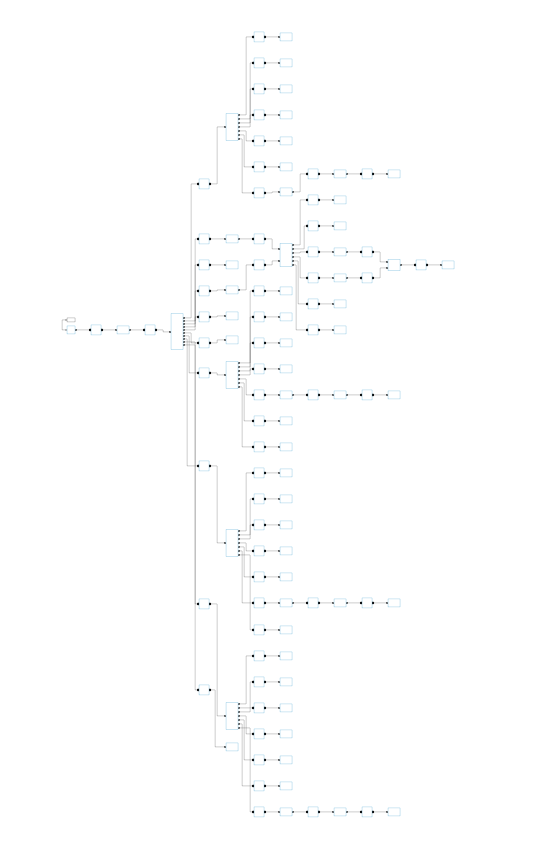在这里补充一个小插曲，有的朋友在这个过程中运行时是无法显示模型图形，只需要修改一下配置，参考下图设置就行了。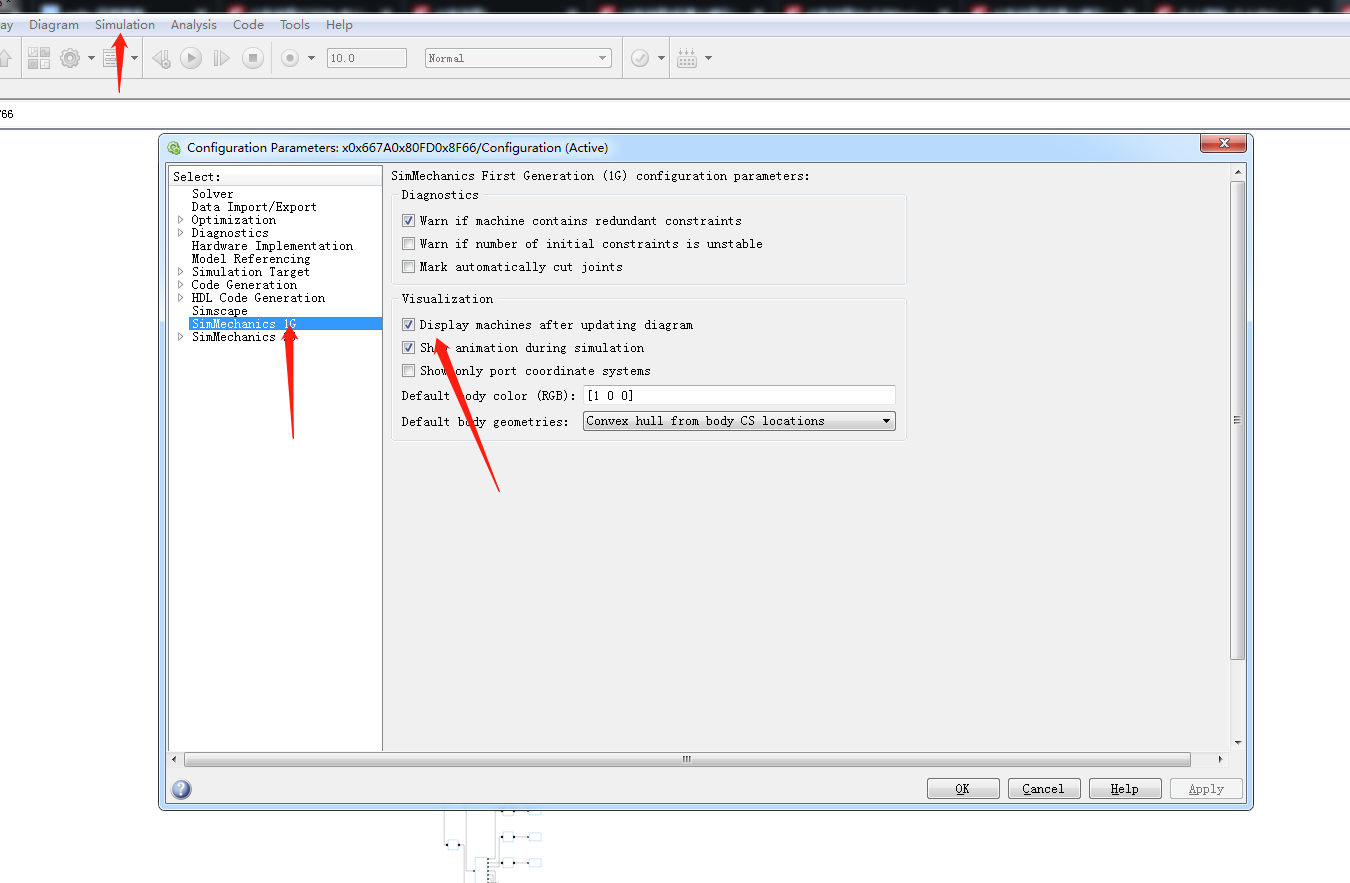最后就可以看到你想要的的效果，没想到放在这里面我的这个模型还不错，挺好看的。剩下的仿真就靠你自己了，祝你好运。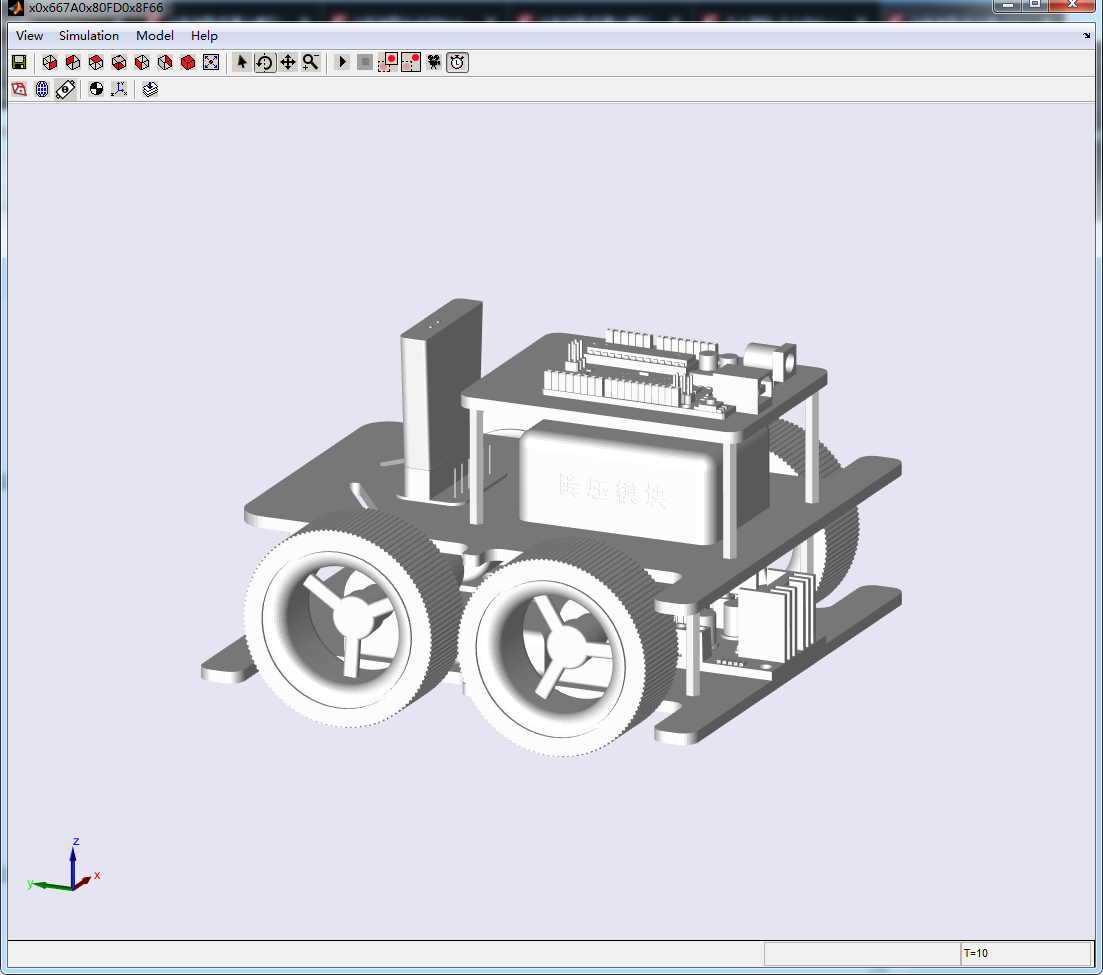3.2 2G操作方法

2G的方法多数和1G相似，就用这句话“同理可证”，不不，把关键步骤安排一下：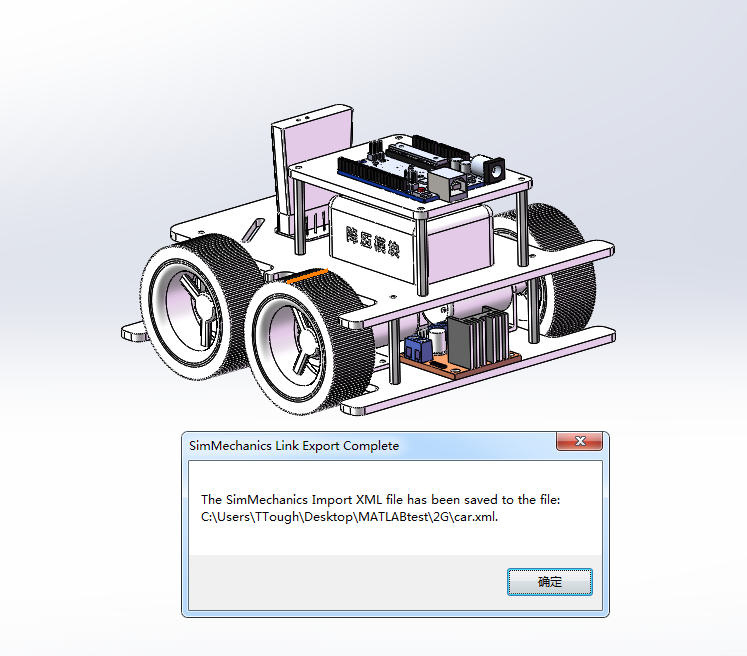硬说区别可以说就在这里，注意看，2G输入的命令是不一样的。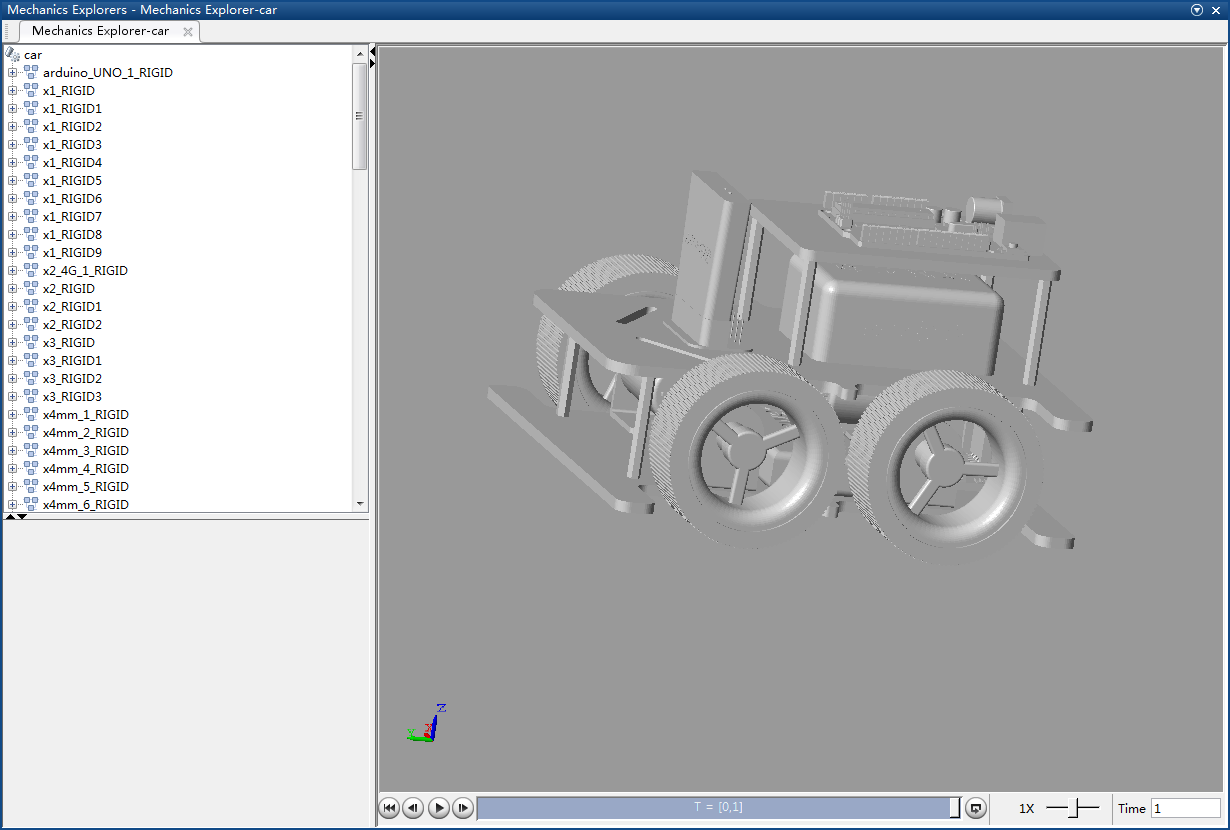最后，大功告成。最后我想说，MATLAB实在太强大了！

感谢大家在这里听我说口水话，后期还会分享一些相关博客，初来乍到，希望各位多多关照。

展开全文solidworks 模型导入matlab
• matlab函数文件，用于读取其他软件生成的3D模型文件obj文件，在matlab中显示模型图像
• MATLAB中建立一个脚本show3Dmat.m文件...load('E:\博士\深度学习与三维重建\代码实现\3DRecGAN\X_Y_pred_01_0000.mat'); %查看维度 %w=size(X_test); %8*64*64*64 a=X_test(1,:,:,:); %取出第一个样本,1*64*64*64...

在MATLAB中建立一个脚本show3Dmat.m文件，编写代码：

clc;
clear;
%查看维度
%w=size(X_test);  %8*64*64*64
a=X_test(1,:,:,:);   %取出第一个样本,1*64*64*64
b=squeeze(a);   %去除第一列,64*64*64

展开全文• matlab打开三维模型数据，读入obj格式人脸数据并用GUI界面显示出来matlab
• 前言:在地球物理勘探,流体空间分布等多种场景中,定位空间点P(x,y,x)的物理属性值Q,并绘制三维空间分布图,对我们洞察空间场景有十分重要的意义. 1. 三维立体图的基本要件: 全空间网格化 网格节点的物理属性值 2.数据...
• 读取 tif文件并绘成三维图，用Matlab实现，是一个很简单有效的代码哟
• matlab旋转矩阵实战： ①点云，按照旋转矩阵后旋转（mesh的话（例如.obj），顶点v按照这个旋转，再把原face加入到旋转后的顶点结果中就行了） （本文matlab2017才可以读取.pcd文件,也可以读入普通.xyz，.txt...
• matlab中我么可以调用mesh(x,y,z)函数来产生三维图像。首先，我们用z=cos(x)sin(y)在-2pi ≤x,y≤ 2pi内的图像来看看:[x,y] = meshgrid(-2*pi:0.1:2*pi);z = cos(x).*sin(y);mesh(x,y,z),xlabel('x'),ylabel('y'),...
• 256028/256028/model3d/256028/model3d/@model3d/256028/model3d/@model3d/center_of_mass.m256028/model3d/@model3d/greyscale.m256028/model3d/@model3d/layer_names.m256028/model3d/@model3d/magnify.m256028/mo...
• MATLAB中建立一个脚本show3...fileID= fopen('E:\博士\深度学习与三维重建\代码实现\voxel_grids_64\chair_0890_0_0_1_062_049_042.txt','r'); %txt文件读成三维元胞数组cell形式 A = textscan(fileID,'%d %d ...
• function surf2stl(filename,x,y,z,mode)%SURF2STL Write STL file from surface data.% SURF2STL('filename',X,Y,Z) writes a stereolithography (STL) file% for a surface with geometry defined by three ...
• 下载链接：Wavefront OBJ toolbox - File Exchange - MATLAB Central​ww2.mathworks.cn函数说明：The function read_wobj allow Wavefront object files including material MTL files to be read into a ...
• 展开全部1、首先打开复matla软件，准制备好要做三维bai图的数据，有XYZ和属性du数据。2、根据数zhi据分布确定各dao点之间的间隔，并使用griddata命令将属性数据也进行插值，这里仅作为例子，我们使用默认的插值方法...
• 本文是博客文章：《MATLAB数学建模：数据图形可视化》的第五章：三维绘图函数部分。数据可视化 数学建模 matlab 数据分析solidworks matlab
• 三维地形图建模仿真与Matlab之间的数据交换

千次阅读 多人点赞 2020-06-20 08:46:51
很多小伙伴在进行科研工作中会需要用到三维地形图，如果用Matlab生成复杂的地形图会很难操作。 而我们很容易在数据库找到想要的DEM（数字高程模型）但下载高程图几乎都要收费，如何对获得的高程图进行操作及处理，...数据建模 matlab
• 答：x=1:10 x = 1 2 3 4 5 6 7 8 9 10 >> y=1:10 y = 1 2 3 4 5 6 7 8 9 10 >> z=1:10 z = 1 2 3 4 5 6 7 8 9 10 >... 此处还有其它函数可以画三维图谱，答：三维曲线绘图 t=(0:0...
• (3)绘制大端、小端齿廓渐开线。MATLAB软件不仅擅长处理数组的各种运算，而且其图形显示功能强大，适合于弧齿锥齿轮的渐开线的建立。因此将如下数据输入到MATLAB中，绘制渐开线：将MATLAB生成的渐开线导人...② 打开S...
• 平面曲线按曲率上色（曲线第三维用颜色表示） 先上效果图 正弦曲线按曲率上色 自由曲线按误差上色 自由曲面按误差上色 曲线第三维用颜色表示实现方法： x=linspace(-10,10,100); y=sin(x); y(end)=NaN; z=abs(sin...matlab
• 方法步骤1、这里小编以常用的三维建模软件SolidWorks为例，将SolidWorks中建立的三维实体模型导入ANSYS(在这里做了去轴处理)。按照Parasolid(*.x_t)格式导入到ANSYS后，模型只显示点、线和面，没...
• 对机械手进行建模采用自底向上的方式进行，即先依据相关零部件的结构和尺寸建立起三维模型，再利用Solidworks的装配体建模，按照各零部件之间的装配以及约束关系逐个进行组装，最后建立虚拟样机。对零部件的精确建模...
• 基于MATLAB的线激光三维彩色扫描仪

万次阅读 多人点赞 2019-08-07 22:30:04
但是由于我也是为了简单的模型设计，项目本身并没有实际参数的要求，故实际测量等对有些变量并没有很好地测量与控制，这并不影响我们对线激光三维成像原理与方法的理解；实际来看实验效果依旧不错，大家可以根据实际...三维扫描 三维重建 结构光三维扫描
• 【2018年最新整理】MATLAB论文数学建模对MATLAB的总结 -----------------------------------------------------2数学建模序言 ------------------------------------------------------3案例背景 ------------------...
• 一些可用于点云处理及三维重建的常用模型mat文件，mat使用matlab打开三维数据格式。 Some common model mat files that can be used for point cloud processing and 3D reconstruction are opened with MATLAB ......

matlab打开三维模型matlab 订阅